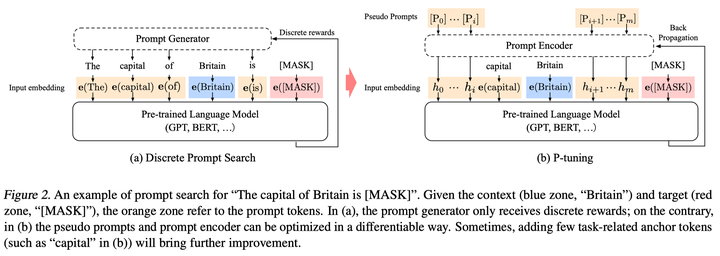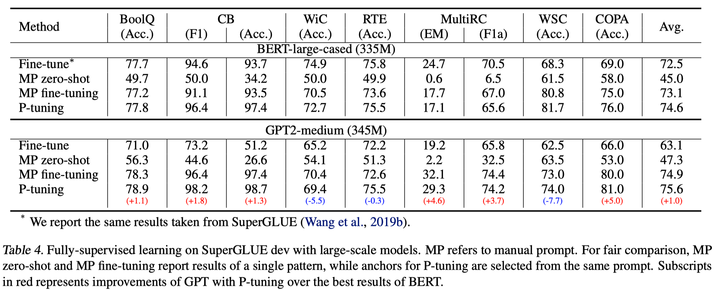Prompt-based Language Models

Introduction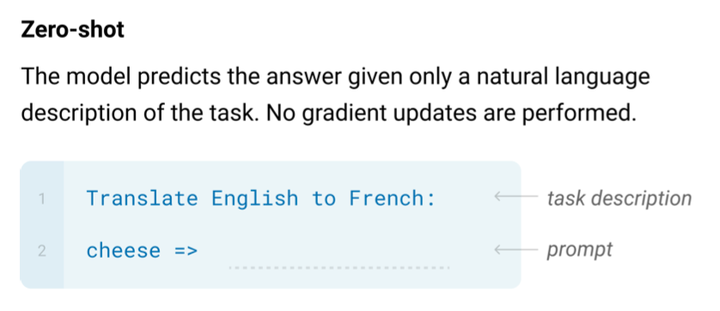Exploiting Cloze Questions for Few Shot Text Classiﬁcation and Natural Language Inference

PET 来自 2020 年的论文（已发表在 EACL 2021）《Exploiting Cloze Questions for Few Shot Text Classiﬁcation and Natural Language Inference》，其中介绍了一种基于模版和词遮盖将文本分类任务转换为完形填空（cloze）任务的半监督训练方法，仅使用 RoBERTa-base 模型就在多个半监督场景下取得了 SOTA：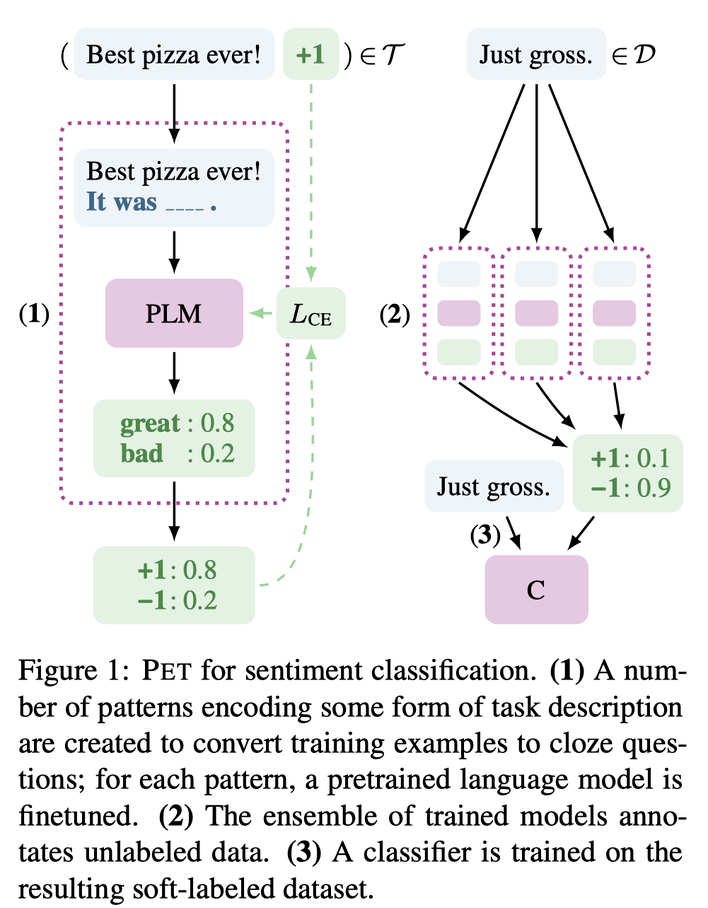1. 首先，针对少量样本设计描述的模版（pattern），如上图中对 “Best pizza ever!” 的情感分类任务，生成一个 “It was ___” 的句子并拼接在原始输入后作为补充；

• 对模版中遮盖的词（即下划线部分），设计候选词对应不同的情感极性（图中 great 对应 positive，bad 对应 negative），然后将模型预测 “great” 的概率作为原来预测 “positive” 的概率，从而将情感分类任务转换为完形填空任务。
• 当然，原文中对 NLI 任务也进行了模版构建，其操作有所不同，在此不展开；
• 注意，完形填空和 MLM 不是一个任务，虽然二者都是词分类任务，但是类别一个是候选词集，一个是模型中全部的词集；
2. 对有标签样本集设计不同的模版，然后对每一个模版，分别训练模型；

• 因为有标签样本比较少，所以训练成本低于全量数据训练一个完整的模型；
• 这里的训练因为是有监督的，所以结合了完形填空的词分类 loss 和 MLM Loss 进行训练：$L=(1-\alpha) \cdot L_{\mathrm{CE}}+\alpha \cdot L_{\mathrm{MLM}}$，其中 MLM loss 占较小比重（1e-4）；
3. 使用上面训练得到的一堆模型，在无标签数据上进行预测，按不同 pattern 的模型 acc 对应权重对所有的预测进行归一化，作为 soft label 蒸馏一个最终模型；

• 这里最终模型并不进行 pattern 的学习；
• 在这里的训练中，不涉及 MLM loss。

• 其实就是进行多代交叉的蒸馏，随机选取每一代的模型为无标签数据进行标记，并基于此进一步训练下一代模型；
• 最终和 PET 一样，用不同模型标注的无标签数据进行预测，蒸馏一个统一的模型。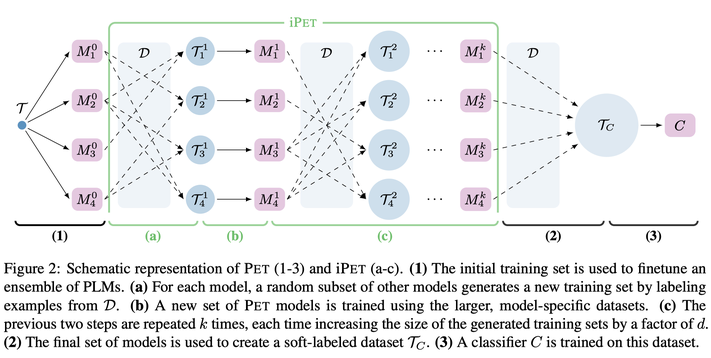• 情感分类（Yelp）：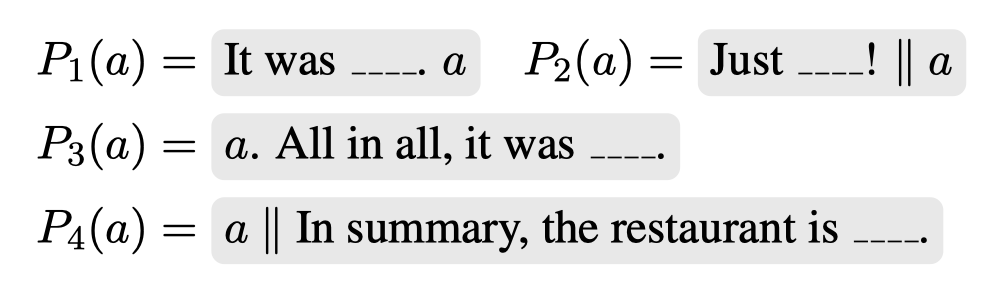• 文本蕴含（MNLI）：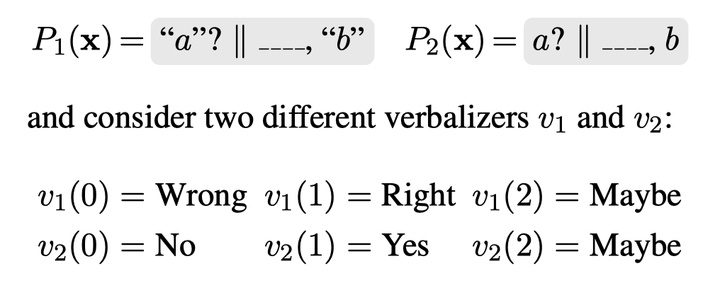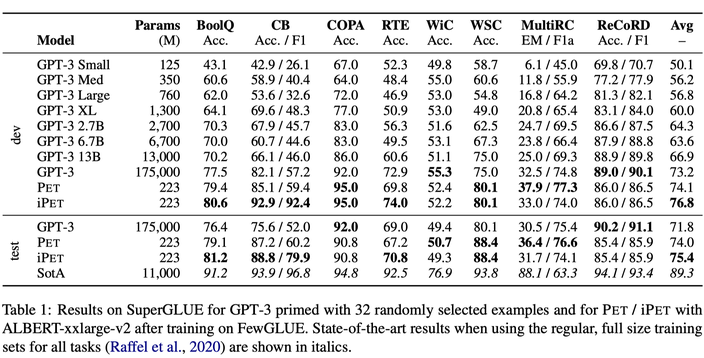_中文实验_

自动构建Prompt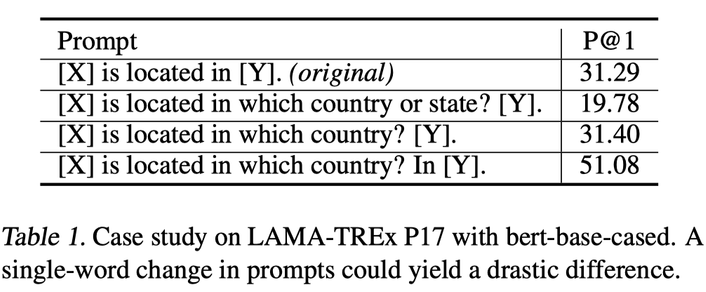LM Prompt And Query Archive

1. Mining-based Generation：基于远程监督的假设（即，出现相同实体对的句子表达相同的关系），在 Wikipedia sentence 中寻找包含头尾实体 h、t 的句子，然后进一步提出了两种 prompt 抽取方法：

• Middle-word Prompts：对于 h、t 中间包含文本的句子形式，将 h、t 中间的文本当作 prompt；
• Dependency-based Prompts：对于其他句子，使用句法依赖解析来提取 h 和 t 最短的路径，并将路径上的词作为 prompt。
2. Paraphrasing-based Generation：类似查询拓展技术，在保持原 prompt 语义同时增加词汇的多样性。这种方法依赖反向翻译（back-translation），即翻译到另一种语言再翻译回来，构成多个样本后根据往返概率（round-trip probability）筛选 prompt。

• Top-1 Prompt Selection：就是用 LM 测一测看看效果，取 acc 最高的 prompt；
• Rank-based Ensemble：除了 Top-1 方案，有时候需要保持多个模版来增强效果，即选取前 K 个模版；
• Optimized Ensemble：通过 LM 的预测为不同的 prompt 赋权。

AUTOPROMPT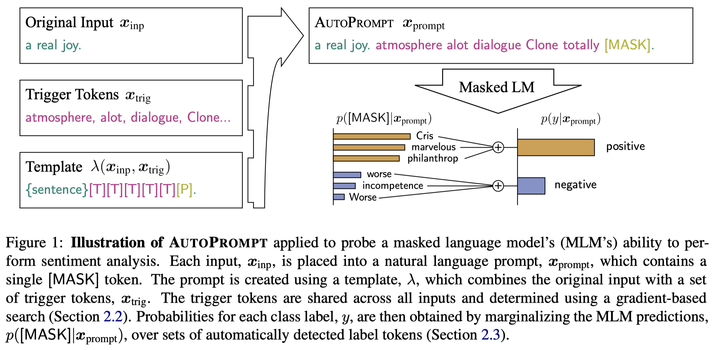AutoPrompt根据模板$\lambda$，将原始任务输入$x$与一系列触发令牌$x_{trig}$相结合，构建一个提示符。注意触发器令牌在所有输入中共享，因此普遍有效。

AutoPrompt使用梯度搜索机制来寻找触发词$x_{trig}$，本质上是计算监督损失的一阶导数：$$e_{\mathrm{trig}}^{(t+1)}=\arg \min _{e \in \mathcal{V}}\left[e-e_{\mathrm{trig}}^{(t)}\right]^{\top} \nabla_{e_{\mathrm{trig}}^{(t)}} \mathcal{L}$$

$$p\left(y \mid \boldsymbol{x}_{\text {prompt }}\right)=\sum_{w \in \mathcal{V}_{y}} p\left([\mathrm{MASK}]=w \mid \boldsymbol{x}_{\text {prompt }}\right)$$

Better Few-shot Fine-tuning of Language Models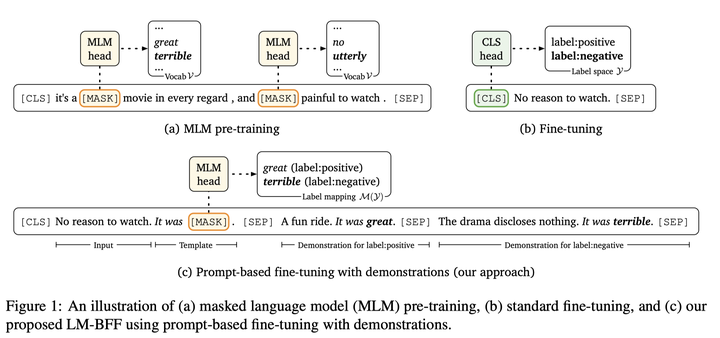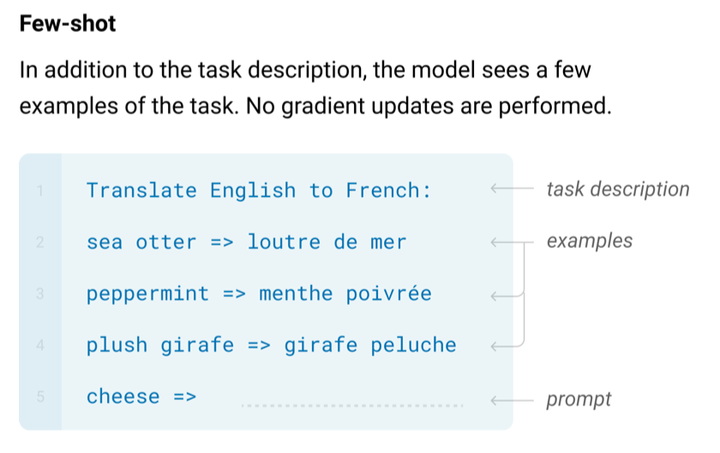LM-BFF包含两个方面，一是自动搜索标签词和模板，一是自适应引入Demonstration。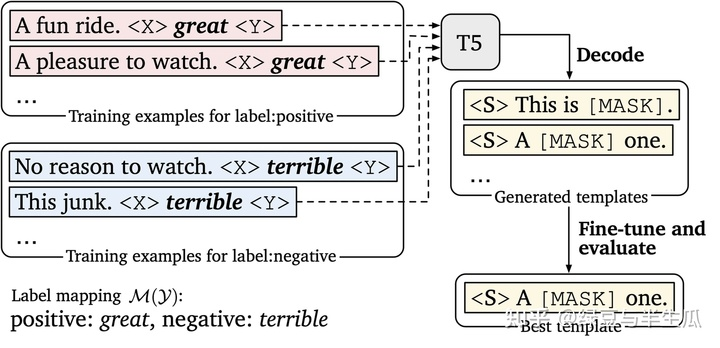• 在训练和推理期间，我们从训练集中为每个类随机抽取一个样本并将它们连接起来。对于推理，我们对抽取多组demonstration，并在最后对结果进行集成。
• 我们只采样与输入密切相关的demonstration。例如，如果输入是电影评论，就对电影评论进行采样，而不是餐厅评论。我们采用 SBERT ( Reimers and Gurevych, 2019 ) 对句子进行编码，计算输入与训练集中所有样本之间的余弦相似度，然后仅从前 50% 的样本中进行采样。

• 可以看出 prompt FT(auto) + demonstrations > prompt FT(auto) > prompt FT(man) > FT；
• 但是全量数据 FT 还是比不过。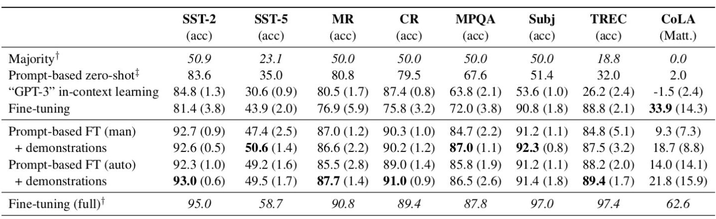Prefix-Tuning: Optimizing Continuous Prompts for Generation

1. 2021.1

Smart prompt design essentially produces efficient context that can lead to desired completion.

2. Prefix-Tuning指在输入序列(命名为prefix)的开头指定少量可训练的参数来控制LM， [prefix;x;y]。Let $P_{idx}$ be a set of prefix indices and dim($h_{i}$) be the embedding size. The prefix parameters $P_{\theta}$ has the dimension $|P_{idx}| \times dim(h_{i})$ and the hidden state takes the form:$$h_{i}=\left\{\begin{array}{ll} P_{\theta}[i,:], & \text { if } i \in \mathcal{P}_{\text {idx }} \\ \operatorname{LM}_{\phi}\left(z_{i}, h_{<i}\right), & \text { otherwise } \end{array}\right.$$
3. 注意训练时预训练模型的参数固定：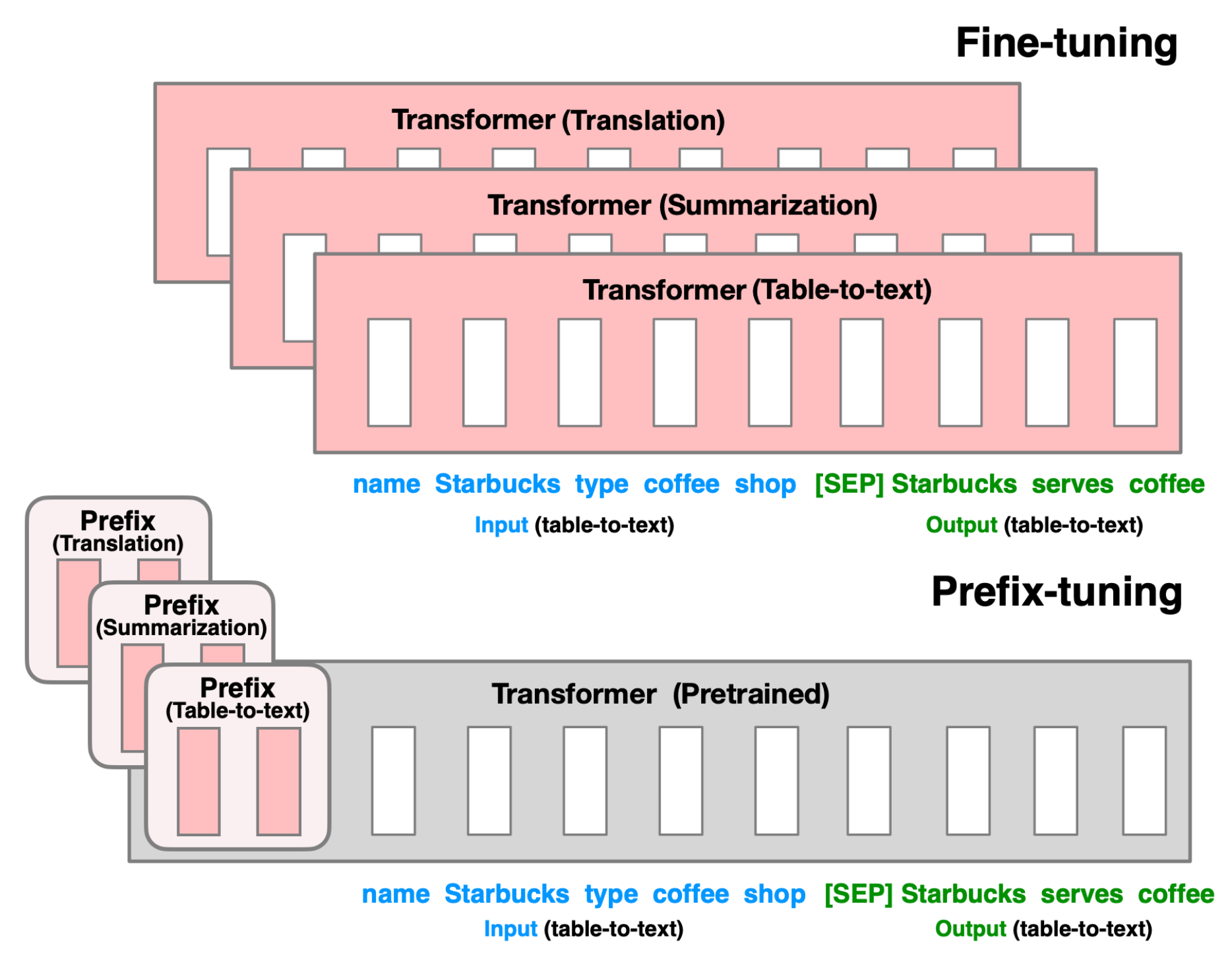4. 实验结果：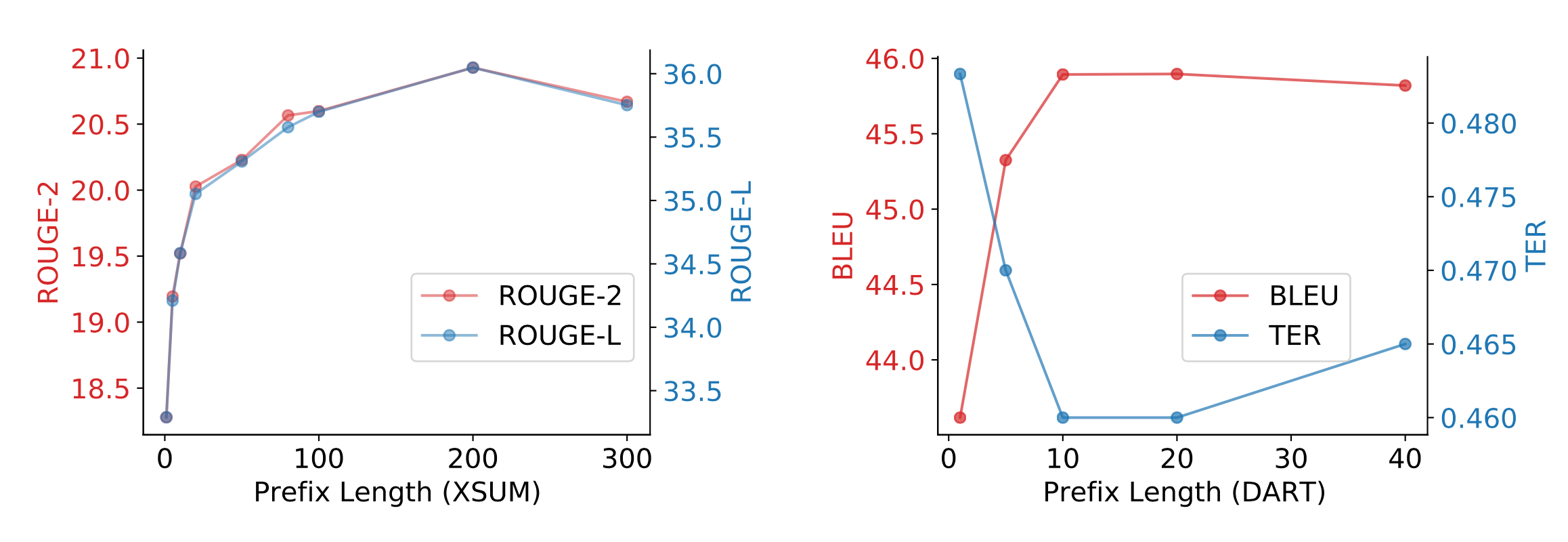5. 结论：

Fine-tuned models achieve better task performance but they can fail in the low data regime. Both AutoPrompt and Prefix-Tuning were found to outperform fine-tuning in the regime where the training dataset is small (i.e. 10^2 − 10^3 samples). As an alternative to fine-tuning, prompt design or learning the context embedding is much cheaper. AutoPrompt improves the accuracy for sentiment classification a lot more than manual prompts and achieves similar performance as linear probing. For the NLI task, AutoPrompt obtains higher accuracy than linear probing. It is able to retrieve facts more accurately than manual prompts too. In low data regime, Prefix-Tuning achieves performance comparable with fine-tuning on table-to-text generation and summarization.

P-tuning